# AP Chemistry : Reaction Types

## Example Questions

### Example Question #11 : Identifying And Defining Acids And Bases

Which of the following is a pairing between an acid and its conjugate base?

PO4, PO3

H2O, COOH

H2CO3, CO2

H3O+, H20

H3O+, H20

Explanation:

The conjugate base of an acid is the same formula, minus one proton. The only option that fits this description is the one that includes the hydronium ion (H3O+) and water (H2O).

### Example Question #12 : Identifying And Defining Acids And Bases

Under which classification(s) of acid does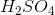fall?

Brønsted-Lowry acid

All of these

Strong acid

Lewis acid

Arrhenius acid

All of these

Explanation:

Every Lewis acid is also a Brønsted-Lowry acid, and every Brønsted-Lowry acid is an Arrhenius acid; thus, H2SO4 is all three, since it is an Arrhenius acid: (it dissolves in water to produce a proton). Sulfuric acid is also considered a strong acid, as it full dissociates in water.

### Example Question #11 : Identifying And Defining Acids And Bases

In the following reaction, which is the conjugate acid?

HCO3– + HCl → H2CO3 + Cl–

HCl

H2CO3

Cl–

HCO3–

H2CO3

Explanation:

Conjugate acid has one more H+ than the compound with which it is being compared. Thus, H2CO3 is the conjugate acid of HCO3–.

### Example Question #61 : Reaction Types

Which of the following is not true of a neutralization reaction?

It is a specific double-displacement reaction.

There is a reaction between acid and base.

It has 2 reactants and 2 products.

There is a reaction between salt and water.

There is a reaction between salt and water.

Explanation:

The PRODUCTS of a neutralization reactions are salt and water, not the reactants. The rest of the options all correctly pertain to neutralization reactions.

### Example Question #62 : Reaction Types

Which of the following chemical groups is expected to be found in a base?

Hydrogen

Bicarbonate

Hydroxide

Chloride

Carboxyl

Hydroxide

Explanation:

Bases can be defined as species that quench hydrogen ions from a solution. A hydroxide ion and a hydrogen ion combine to form water in solution. Recall that basis solutions range in pH from about 7 to 14.

### Example Question #471 : Ap Chemistry

Which of the following groups is expected to be present in an acid?

Carboxyl

Bicarbonate

Hydroxide

Hydrogen

Chloride

Hydrogen

Explanation:

Acids can be defined as species that donate hydrogen ions to solutions. If there is a hydrogen group on a molecule, it is possible that it may be donated to the solution, which will result in a decrease in pH.

### Example Question #61 : Acid Base Reactions

Which compound can be both a Bronsted-Lowry acid and Bronsted-Lowry base?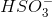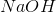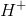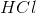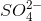Explanation:

The Bronsted-Lowry definition of an acid is a substance that can donate a hydrogen ion and forms its conjugate base; a Bronsted-Lowry base accepts a hydrogen ion and forms its conjugate acid. Thus we are looking for a substance that can either donate or accept a hydrogen ion (amphoteric). Bisulfite may give up a proton to become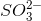, a Bronsted-Lowry base. It acts as an acid as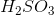, which can donate up to two hydrogens.

### Example Question #61 : Reaction Types

Which of the following is a Lewis acid, but not a Bronsted-Lowry acid?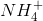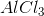Explanation:

For this question, we'll need to understand the different definitions of an acid in order to answer it. There are three definitions of acids that are important to know.

1. Arrhenius acids

These are compounds that, when added to water, increase the concentration ofions present in solution.

2. Bronsted-Lowry acids

These are any acid that can release, even while not in water.

3. Lewis acids

This is the most general definition of acids. It is any compound that can accept a lone electron pair.

Lewis acids are the most general kind of acids, meaning that any acid that is Bronsted-Lowry or Arrhenius will also be a Lewis acid. However, the reverse is not true. Not all Lewis acids will fall under the category of Bronsted-Lowry or Arrhenius.

The correct answer in this question is aluminum chloride. We can see that, based on aluminum's position in the periodic table, it has three valence electrons in its outer shell. Each of these electrons is tied up in a shared bond with a chloride. This means that the aluminum in aluminum chloride has six valence electrons. However, since aluminum has a maximum capacity of eight valence electrons, it has room for two more. This vacancy allows the aluminum component of aluminum chloride to accept an electron pair from any sort of electron donor. Thus, aluminum chloride qualifies as a Lewis acid. However, aluminum chloride has no way of producing. Consequently, it is neither a Bronsted-Lowry acid nor is it an Arrhenius acid.

### Example Question #1 : Strong And Weak Acids And Bases

Which of the following is a strong acid?

Acetic acid

Nitric acid

Formic acid

Lactic acid

Hydroflouric acid

Nitric acid

Explanation:

This question is simply testing your memorization of strong and weak acids. Of the list, you should recognize that nitric acid is the only strong acid, and the rest of the choices are weak.

### Example Question #1 : Strong And Weak Acids And Bases

Which of the following is what determines the strength of an acid?

The Ka

Its physical state

The Kb

Electronegativity values

How many bonds the central atom makes